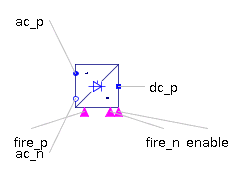Reluctance Rotor - MapleSim Help

QuasiStatic SM Reluctance Rotor

Synchronous induction machine with reluctance rotor and damper cageDescription

The QuasiStatic SM Reluctance Rotor component models a multiphase synchronous induction machine with reluctance rotor and damper cage.

Resistances and stray inductances of the machine refer to an $m$-phase stator. The symmetry of the stator and the supply are assumed. Rotor asymmetries are modeled resistances and stray inductances in the d- and q-axis.

The following losses are modeled:

 • heat losses in the temperature dependent stator winding resistances
 • heat losses in the temperature dependent damper cage resistances
 • friction losses
 • core losses (only eddy current losses, no hysteresis losses)Connections

 Name Description Modelica ID $\mathrm{flange}$ Shaft flange $\mathrm{support}$ Support at which the reaction torque is acting support ${\mathrm{plug}}_{\mathrm{sp}}$ Positive plug of stator plug_sp ${\mathrm{plug}}_{\mathrm{sn}}$ Negative plug of stator plug_sn $\mathrm{thermalPort}$ Thermal port of induction machines thermalPortParametersGeneral Parameters

 Name Default Units Description Modelica ID ${f}_{s,\mathrm{nom}}$ $50$ $\mathrm{Hz}$ Nominal frequency fsNominal ${J}_{r}$ $0.29$ $\mathrm{kg}{m}^{2}$ Rotor moment of inertia Jr ${J}_{s}$ ${J}_{r}$ $\mathrm{kg}{m}^{2}$ Stator moment of inertia Js ${L}_{\mathrm{s0}}$ ${L}_{s\sigma }$ $H$ Stator zero inductance Lszero $m$ $3$ Number of stator phases m $p$ $2$ Number of pole pairs (integer) p ${T}_{r,\mathrm{oper}}$ $293.15$ $K$ Operational temperature of (optional) damper cage TrOperational ${T}_{s,\mathrm{oper}}$ $293.15$ $K$ Operational temperature of stator resistance TsOperational Effective Stator Turns $1$ Effective number of stator turns effectiveStatorTurns Use Support Flange $\mathrm{false}$ True (checked) means stator support is enabled useSupport Use Thermal Port $\mathrm{false}$ True (checked) means heat port is enabled useThermalPortFriction Losses

 Name Default Units Description Modelica ID ${P}_{\mathrm{ref}}$ $0$ $W$ Reference friction losses PRef ${\omega }_{\mathrm{ref}}$ ${\omega }_{\mathrm{nom}}$ $\frac{\mathrm{rad}}{s}$ Reference angular velocity wRef ${p}_{\omega }$ $2$ $V$ Exponent of friction power_w

See Friction for details of the friction loss model.Stator Core Losses

 Name Default Units Description Modelica ID ${P}_{\mathrm{ref}}$ $0$ $W$ Reference rotor core losses PRef ${V}_{\mathrm{ref}}$ $V$ Reference RMS voltage VRef ${\omega }_{\mathrm{ref}}$ $2\pi {f}_{s,\mathrm{nom}}$ $\frac{\mathrm{rad}}{s}$ Reference angular velocity wRef

See Core for details of the core loss model.Name Default Units Description Modelica ID ${I}_{\mathrm{ref}}$ ${I}_{{a}_{\mathrm{nom}}}$ $W$ Reference RMS current IRef ${P}_{\mathrm{ref}}$ $0$ $W$ Reference friction losses PRef ${\omega }_{\mathrm{ref}}$ ${\omega }_{\mathrm{nom}}$ $\frac{\mathrm{rad}}{s}$ Reference angular velocity wRef ${p}_{\omega }$ $1$ $V$ Exponent of stray load loss power_wNominal Resistances And Inductances Parameters

 Name Default Units Description Modelica ID ${\mathrm{\alpha }}_{r}$ $0$ $\frac{1}{K}$ Temperature coefficient of damper resistances in d- and q-axis alpha20r ${\mathrm{\alpha }}_{s}$ $0$ $\frac{1}{K}$ Temperature coefficient of stator resistance at 20 degC alpha20s ${L}_{\mathrm{md}}$  $H$ Main field inductance in d-axis Lmd ${L}_{\mathrm{mq}}$  $H$ Main field inductance in q-axis Lmq ${L}_{r\sigma d}$  $H$ Rotor leakage inductance, d-axis, w.r.t. stator side Lrsigmad ${L}_{r\sigma q}$ ${L}_{r\sigma d}$ $H$ Rotor leakage inductance, q-axis, w.r.t. stator side Lrsigmaq ${L}_{s\sigma }$  $H$ Stator stray inductance per phase Lssigma ${R}_{\mathrm{rd}}$ $0.04$ $\mathrm{\Omega }$ Rotor resistance, d-axis, w.r.t. stator side Rrd ${R}_{\mathrm{rq}}$ ${R}_{\mathrm{rd}}$ $\mathrm{\Omega }$ Rotor resistance, q-axis, w.r.t. stator side Rrq ${R}_{s}$ $0.03$ $\mathrm{\Omega }$ Warm damper resistance in q-axis Rs ${T}_{r,\mathrm{ref}}$ $293.15$ $K$ Reference temperature of damper resistances in d- and q-axis TrRef ${T}_{s,\mathrm{ref}}$ $293.15$ $K$ Reference temperature of stator resistance TsRef Use Damper Cage $\mathrm{true}$ True (checked) means damper cage is enabled useDamperCage

 $\frac{0.4615493349}{{f}_{{s}_{\mathrm{nom}}}}$

 $\frac{0.1432394488}{{f}_{{s}_{\mathrm{nom}}}}$

 $\frac{0.025}{\mathrm{\pi }{f}_{{s}_{\mathrm{nom}}}}$

 $\frac{0.01591549431}{{f}_{{s}_{\mathrm{nom}}}}$Modelica Standard Library The component described in this topic is from the Modelica Standard Library. To view the original documentation, which includes author and copyright information, click here.